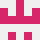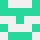# Pandoc lua 过滤器，将图片标题移到文档末尾

\begin{figure}
\includegraphics[width=1\linewidth]{figure1} \caption{Figure 1. Caption.}\label{fig:figure1}
\end{figure}


\section*{图表}
\begin{figure}[h!]
\caption{Figure 1. Caption.}
\end{figure}

\begin{figure}[h!]
\caption{Figure 2. Caption.}
\end{figure}


\begin{figure}
\includegraphics[width=1\linewidth]{figure1} \caption{Figure 1. Caption.}\label{fig:figure1}
\end{figure}


function Para (el) print(pandoc.utils.stringify(el)) endstackoverflow用户2777074

\usepackage{figcaps}

2022-02-27 16:11:45stackoverflow用户2425163

local captions = pandoc.List()

function RawBlock (raw)
local caption = raw.text:match('\\caption%{(.*)%}')
if caption then
captions:insert(caption)
end
end

function Pandoc (doc)
-- 将收集到的标题追加到末尾
doc.blocks:insert(captions:map(function(x) return pandoc.Para(x) end))
return doc
end


function Pandoc (doc)
doc.meta.figures = captions:map(function (c)
return pandoc.RawBlock('latex', c)
end)
return doc
end

2022-02-28 14:35:48